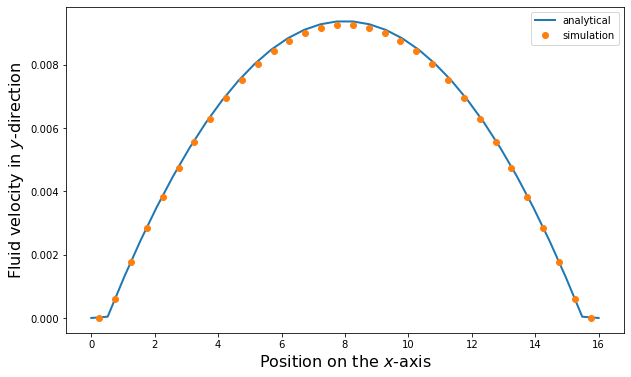# The Lattice-Boltzmann Method in ESPResSo - Part 4¶

## Poiseuille flow in ESPResSo¶

Poiseuille flow is the flow through a pipe or (in our case) a slit under a homogeneous force density, e.g. gravity. In the limit of small Reynolds numbers, the flow can be described with the Stokes equation. We assume the slit being infinitely extended in $y$ and $z$ direction and a force density $f_y$ on the fluid in $y$ direction. No slip-boundary conditions (i.e. $\vec{u}=0$) are located at $x = \pm h/2$. Assuming invariance in $y$ and $z$ direction and a steady state, the Stokes equation is simplified to:

\begin{equation} \mu \partial_x^2 u_y = f_y \end{equation}

where $f_y$ denotes the force density and $\mu$ the dynamic viscosity. This can be integrated twice and the integration constants are chosen so that $u_y=0$ at $x = \pm h/2$ to obtain the solution to the planar Poiseuille flow :

\begin{equation} u_y(x) = \frac{f_y}{2\mu} \left(h^2/4-x^2\right) \end{equation}

We will simulate a planar Poiseuille flow using a square box, two walls with normal vectors $\left(\pm 1, 0, 0 \right)$, and an external force density applied to every node.

### 1. Setting up the system¶

In :
import logging
import sys

import matplotlib.pyplot as plt

In :
import numpy as np

import espressomd
import espressomd.lb
import espressomd.lbboundaries
import espressomd.shapes

logging.basicConfig(level=logging.INFO, stream=sys.stdout)

espressomd.assert_features(['LB_BOUNDARIES_GPU'])

# System constants
BOX_L = 16.0
TIME_STEP = 0.01

system = espressomd.System(box_l=[BOX_L] * 3)
system.time_step = TIME_STEP
system.cell_system.skin = 0.4


#### 1.1 Setting up the lattice-Boltzmann fluid¶

We will now create a lattice-Boltzmann fluid confined between two walls.

In :
# LB parameters
AGRID = 0.5
VISCOSITY = 2.0
FORCE_DENSITY = [0.0, 0.001, 0.0]
DENSITY = 1.5

# LB boundary parameters
WALL_OFFSET = AGRID


Create a lattice-Boltzmann actor and append it to the list of system actors. Use the GPU implementation of LB.

You can refer to section setting up a LB fluid in the user guide.

In :
logging.info("Setup LB fluid.")
lbf = espressomd.lb.LBFluidGPU(agrid=AGRID, dens=DENSITY, visc=VISCOSITY, tau=TIME_STEP,
ext_force_density=FORCE_DENSITY)

INFO:root:Setup LB fluid.


Create a LB boundary and append it to the list of system LB boundaries.

You can refer to section using shapes as lattice-Boltzmann boundary in the user guide.

In :
logging.info("Setup LB boundaries.")
top_wall = espressomd.shapes.Wall(normal=[1, 0, 0], dist=WALL_OFFSET)
bottom_wall = espressomd.shapes.Wall(normal=[-1, 0, 0], dist=-(BOX_L - WALL_OFFSET))

top_boundary = espressomd.lbboundaries.LBBoundary(shape=top_wall)
bottom_boundary = espressomd.lbboundaries.LBBoundary(shape=bottom_wall)


INFO:root:Setup LB boundaries.

Out:
<espressomd.lbboundaries.LBBoundary at 0x7f45b9576770>

### 2. Simulation¶

We will now simulate the fluid flow until we reach the steady state.

In :
logging.info("Iterate until the flow profile converges (5000 LB updates).")
system.integrator.run(5000)

INFO:root:Iterate until the flow profile converges (5000 LB updates).


### 3. Data analysis¶

We can now extract the flow profile and compare it to the analytical solution for the planar Poiseuille flow.

In :
logging.info("Extract fluid velocities along the x-axis")
fluid_positions = np.zeros(lbf.shape)
fluid_velocities = np.zeros(lbf.shape)
for x in range(lbf.shape):
# Average over the nodes in y direction
v = [lbf[x, y, 0].velocity for y in range(lbf.shape)]
fluid_velocities[x] = np.average(v)
fluid_positions[x] = (x + 0.5) * AGRID

def poiseuille_flow(x, force_density, dynamic_viscosity, height):
return force_density / (2 * dynamic_viscosity) * (height**2 / 4 - x**2)

# Note that the LB viscosity is not the dynamic viscosity but the
# kinematic viscosity (mu=LB_viscosity * density)
x_values = np.linspace(0.0, BOX_L, lbf.shape)
HEIGHT = BOX_L - 2.0 * AGRID
# analytical curve
y_values = poiseuille_flow(x_values - (HEIGHT / 2 + AGRID), FORCE_DENSITY,
VISCOSITY * DENSITY, HEIGHT)
# velocity is zero inside the walls
y_values[np.nonzero(x_values < WALL_OFFSET)] = 0.0
y_values[np.nonzero(x_values > BOX_L - WALL_OFFSET)] = 0.0

fig1 = plt.figure(figsize=(10, 6))
plt.plot(x_values, y_values, '-', linewidth=2, label='analytical')
plt.plot(fluid_positions, fluid_velocities, 'o', label='simulation')
plt.xlabel('Position on the $x$-axis', fontsize=16)
plt.ylabel('Fluid velocity in $y$-direction', fontsize=16)
plt.legend()
plt.show()

INFO:root:Extract fluid velocities along the x-axisS. Succi. The lattice Boltzmann equation for fluid dynamics and beyond. Clarendon Press, Oxford, 2001.
 B. Dünweg and A. J. C. Ladd. Advanced Computer Simulation Approaches for Soft Matter Sciences III, chapter II, pages 89–166. Springer, 2009.
 B. Dünweg, U. Schiller, and A.J.C. Ladd. Statistical mechanics of the fluctuating lattice-Boltzmann equation. Phys. Rev. E, 76:36704, 2007.
 P. G. de Gennes. Scaling Concepts in Polymer Physics. Cornell University Press, Ithaca, NY, 1979.
 M. Doi. Introduction to Polymer Physics. Clarendon Press, Oxford, 1996.
 Michael Rubinstein and Ralph H. Colby. Polymer Physics. Oxford University Press, Oxford, UK, 2003.
 Daan Frenkel and Berend Smit. Understanding Molecular Simulation. Academic Press, San Diego, second edition, 2002.
 W. E. Langlois and M. O. Deville. Exact Solutions to the Equations of Viscous Flow. In: Slow Viscous Flow, Springer, Cham, 2014.